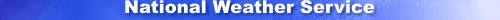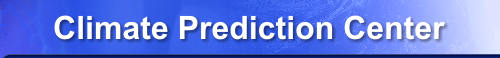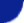Site Map News Organization SearchHOME > Monitoring_and_Data > Oceanic and Atmospheric Data > Reanalysis: Atmospheric Data > wgrib2-set_hex

Introduction

The -set_hex option sets 1 octets (byte) to a a specified hex value. The hex value ranges from 0 to ff.

-set_hex I J K
I = section number = 1..7
J = location in the section = 1..(section length)
K = 00 .. ff
would set
Section I, Octet J to hex value K

-set_hex I J K1:K2:K3:..KN
I = section number = 1..7
J = location in the section = 1..(section length)
Ki = 00 .. ff

Sets Section I, Octet J to K1
Sets Section I, Octet J+1 to K2
etc

-set_hex I J K1K2K3..KN           for v2.0.8+
I = section number = 1..7
J = location in the section = 1..(section length)
Ki = 00 .. ff
Sets Section I, Octet J to K1
Sets Section I, Octet J+1 to K2
etc

Usage

-set_hex  SECTION STARTING_OCTET_LOCATION (I-1):(I-2):..:(I-N)
-set_hex  SECTION STARTING_OCTET_LOCATION (I-1)(I-2)..(I-N)
SECTION=0 .. 7
OCTET_LOCATION = 1..N
I-M = Mth octet as 2 digit hex number

Example

This examples requires wgrib2 v2.0.8+

The file, template_512.grb, was converted from grib1, and the delta-lon, and the extreme latitudes were only to the nearest millidegree (grib1 precision).

wgrib2 template_512.grb -grid
1:0:grid_template=40:winds(N/S):
Gaussian grid: (512 x 256) units 1e-06 input WE:NS output WE:SN
number of latitudes between pole-equator=128 #points=131072
lat 89.463000 to -89.463000
lon 0.000000 to 359.233000 by 0.703000
The grid with full precision can be obtained by using -new_grid, and the contents of sec3 (grid definition) can be obtained by -0xSec 3.
-sh-4.1\$ wgrib2 template_512.grb -new_grid_winds earth -new_grid ncep grid 170 junk
+ wgrib2 template_512.grb -new_grid_winds earth -new_grid ncep grid 170 junk
1:0:d=2016010109:PRES:mean sea level:2390 hour fcst:
-sh-4.1\$ wgrib2 junk -0xSec 3
+ wgrib2 junk -0xSec 3
1:0:Sec3(1..72)=0x0000004803000002000000000028060000000000000000000000000000000000020000000
10000000000ffffffff05551826000000003085551826156a6f6b000aba950000008000
The script to change the precision of the Gaussian grid is given by
sec3='000000480300000200000000002806000000000000000000000000000000000002000000010000000000ffffffff05551826000000003085551826156a6f6b000aba950000008000'
wgrib2 \$1 -set_hex 3 1 "\$sec3" -grid -grib \$1.new

The above script assumes that the size of the original section 3 is greater or equal to the size of the new section 3. If it isn't, you have to use the option -set_sec_size 3 72. Of course, you cannot make arbitrary changes to the grid definition because the number of grid points has to match the grid points in the data section.